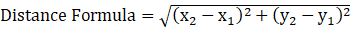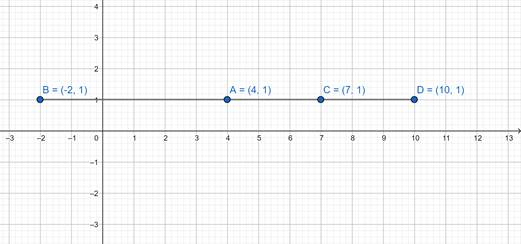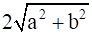### Coordinate Geometry Class 9th Mathematics Term 1 Tamilnadu Board Solution

##### Question 1.The point (–2, 7) lies is the quadrantA. IB. IIC. IIID. IVAnswer:Option A: value to lie in I quadrant, both should be positive. Hence, this is not correct.Option B: value to lie in II quadrant, x–coordinate should be negative and y–coordinate should be positive. Hence, this is correct.Option C: value to lie in III quadrant, both should be negative. Hence, this is not correct.Option D: value to lie in I quadrant, x–coordinate should be positive and y– coordinate should be negative. Hence, this is not correct.Question 2.The point (x, 0) where x < 0 lies onA. OXB. OYC. OX’D. OY’Answer:Option A: point on OX, x–coordinate will be greater than 0 i.e. x > 0.Option B: point on OY, y–coordinate will be greater than 0 i.e. y > 0.Option C: point on OX’, x–coordinate will be lesser than 0 i.e. x < 0.Option D: point on OY’, y–coordinate will be lesser than 0 i.e. y < 0.Question 3.For a point A (a, b) lying in quadrant IIIA. a > 0, b < 0B. a < 0, b < 0C. a > 0, b > 0D. a < 0, b > 0Answer:Option A: point with a > 0, b < 0, lies in the IV quadrant.Option B: point with a < 0, b < 0, lies in the III quadrant.Option C: point with a > 0, b > 0, lies in the I quadrant.Option D: point with a < 0, b > 0, lies in the II quadrant.Question 4.The diagonal of a square formed by the points (1, 0) (0, 1) (–1, 0) and (0, –1) isA. 2B. 4C. √2D. 8Answer:Formula used:Let the points be A (1, 0), B (0, 1), C (–1, 0) and D (0, –1)Distance of diagonal AC⇒ AC = √ ((–1 – 1)2 + ( 0 – 0)2)⇒ AC = √ ((–2)2 + (0)2)⇒ AC =√ (4 + 0)⇒ AC = √ 4⇒ AC = 2∴ Option A is correct.Question 5.The triangle obtained by joining the points A (–5, 0) B (5, 0) and C (0, 6) isA. an isosceles triangleB. right triangleC. scalene triangleD. an equilateral triangleAnswer:Formula used:Let the point be A (–5, 0) B (5, 0) and C (0, 6)Distance of AB⇒ AB = √ (5 – (–5))2 + (0 – 0)2)⇒ AB = √ (5 + 5)2 + (0 – 0)2)⇒ AB = √ ((10)2 + (0)2)⇒ AB = √ (100 + 0)⇒ AB = √ 100 = 10Distance of AC⇒ AC = √ ((0 – (–5))2 + (6 – 0)2)⇒ AC = √ ((5)2 + (6)2)⇒ AC = √ (25 + 36)⇒ AC = √ 61Distance of BC⇒ BC = √ ((0 – 5)2 + (6 – 0)2)⇒ BC = √ ((–5)2 + (6)2)⇒ BC = √ (25 + 36)⇒ BC = √ 61We notice that BC = AC = √ 61∴ Points A, B and C are coordinates of an isosceles triangle.Question 6.The distance between the points (0, 8) and (0, –2) isA. 6B. 100C. 36D. 10Answer:Formula used:Let the point be A (0, 8) and B (0, –2)Distance of AB⇒ AB = √ (0 – 0)2 + (–2 – 8)2)⇒ AB = √ ((0)2 + (–10)2)⇒ AB = √ (0 + 100)⇒ AB = √ 100⇒ AB = 10∴ , Option B is correct.Question 7.(4, 1), (–2, 1), (7, 1) and (10, 1) are pointsA. on x–axisB. on a line parallel to x–axisC. on a line parallel to y–axisD. on y–axisAnswer:Question 8.The distance between the points (a, b) and (–a, –b) isA. 2aB. 2bC. 2a + 2bD.Answer:Formula used:Let the point be A (a, b) and B (–a, –b)Distance of AB⇒ AB = √ (–a – a)2 + (–b – b)2)⇒ AB = √ ((–2a)2 + (–2b)2)⇒ AB = √ (4a2 + 4b2)⇒ AB = √ 4(a2 + b2)⇒ AB = 2√ (a2 + b2)∴ Option D is correct.Question 9.The point which is on y–axis with ordinate –5 isA. (0, −5)B. (−5, 0)C. (5, 0)D. (0, 5)Answer:For any point on y–axis, x–coordinate is 0.∴ the point is (0, –5).Question 10.The relation between p and q such that the point (p, q) is equidistant from (–4, 0) and (4, 0) isA. p = 0B. q = 0C. p + q = 0D. p + q = 8Answer:Formula used:Let the point be A (p, q), B (–4, 0) and C (4, 0)Distance of AB⇒ AB = √ (–4 – p)2 + (0 – q)2)⇒ AB = √ ((–4 – p)2 + (–q)2)⇒ AB = √ (16 + p2 + 8p + q2)Distance of AC⇒ AC = √ (4 – p)2 + (0 – q)2)⇒ AC = √ ((4 – p)2 + (–q)2)⇒ AC = √ (16 + p2 – 8p + q2)i.e. AB = AC (Given)⇒ 16 + p2 + 8p + q2 = 16 + p2 – 8p + q2Squaring both sides⇒ 16 + p2 + 8p + q2 = 16 + p2 – 8p + q2⇒ 16 + p2 + 8p + q2 – 16 – p2 + 8p – q2 = 0 …⇒ 16 p = 0⇒ p = 0∴ Option A is correct.

PDF FILE TO YOUR EMAIL IMMEDIATELY PURCHASE NOTES & PAPER SOLUTION. @ Rs. 50/- each (GST extra)

HINDI ENTIRE PAPER SOLUTION

MARATHI PAPER SOLUTION

SSC MATHS I PAPER SOLUTION

SSC MATHS II PAPER SOLUTION

SSC SCIENCE I PAPER SOLUTION

SSC SCIENCE II PAPER SOLUTION

SSC ENGLISH PAPER SOLUTION

SSC & HSC ENGLISH WRITING SKILL

HSC ACCOUNTS NOTES

HSC OCM NOTES

HSC ECONOMICS NOTES

HSC SECRETARIAL PRACTICE NOTES

# 2019 Board Paper Solution

HSC ENGLISH SET A 2019 21st February, 2019

HSC ENGLISH SET B 2019 21st February, 2019

HSC ENGLISH SET C 2019 21st February, 2019

HSC ENGLISH SET D 2019 21st February, 2019

SECRETARIAL PRACTICE (S.P) 2019 25th February, 2019

HSC XII PHYSICS 2019 25th February, 2019

CHEMISTRY XII HSC SOLUTION 27th, February, 2019

OCM PAPER SOLUTION 2019 27th, February, 2019

HSC MATHS PAPER SOLUTION COMMERCE, 2nd March, 2019

HSC MATHS PAPER SOLUTION SCIENCE 2nd, March, 2019

SSC ENGLISH STD 10 5TH MARCH, 2019.

HSC XII ACCOUNTS 2019 6th March, 2019

HSC XII BIOLOGY 2019 6TH March, 2019

HSC XII ECONOMICS 9Th March 2019

SSC Maths I March 2019 Solution 10th Standard11th, March, 2019

SSC MATHS II MARCH 2019 SOLUTION 10TH STD.13th March, 2019

SSC SCIENCE I MARCH 2019 SOLUTION 10TH STD. 15th March, 2019.

SSC SCIENCE II MARCH 2019 SOLUTION 10TH STD. 18th March, 2019.

SSC SOCIAL SCIENCE I MARCH 2019 SOLUTION20th March, 2019

SSC SOCIAL SCIENCE II MARCH 2019 SOLUTION, 22nd March, 2019

XII CBSE - BOARD - MARCH - 2019 ENGLISH - QP + SOLUTIONS, 2nd March, 2019

# HSCMaharashtraBoardPapers2020

(Std 12th English Medium)

HSC ECONOMICS MARCH 2020

HSC OCM MARCH 2020

HSC ACCOUNTS MARCH 2020

HSC S.P. MARCH 2020

HSC ENGLISH MARCH 2020

HSC HINDI MARCH 2020

HSC MARATHI MARCH 2020

HSC MATHS MARCH 2020

# SSCMaharashtraBoardPapers2020

(Std 10th English Medium)

English MARCH 2020

HindI MARCH 2020

Hindi (Composite) MARCH 2020

Marathi MARCH 2020

Mathematics (Paper 1) MARCH 2020

Mathematics (Paper 2) MARCH 2020

Sanskrit MARCH 2020

Sanskrit (Composite) MARCH 2020

Science (Paper 1) MARCH 2020

Science (Paper 2)

Geography Model Set 1 2020-2021

MUST REMEMBER THINGS on the day of Exam

Are you prepared? for English Grammar in Board Exam.

Paper Presentation In Board Exam

How to Score Good Marks in SSC Board Exams

Tips To Score More Than 90% Marks In 12th Board Exam

How to write English exams?

How to prepare for board exam when less time is left

How to memorise what you learn for board exam

No. 1 Simple Hack, you can try out, in preparing for Board Exam

How to Study for CBSE Class 10 Board Exams Subject Wise Tips?

JEE Main 2020 Registration Process – Exam Pattern & Important Dates

NEET UG 2020 Registration Process Exam Pattern & Important Dates

How can One Prepare for two Competitive Exams at the same time?

8 Proven Tips to Handle Anxiety before Exams!